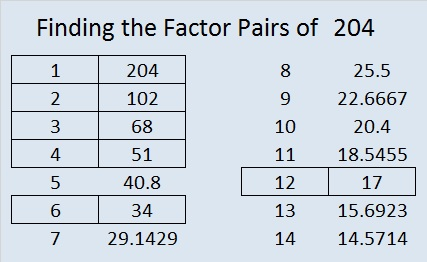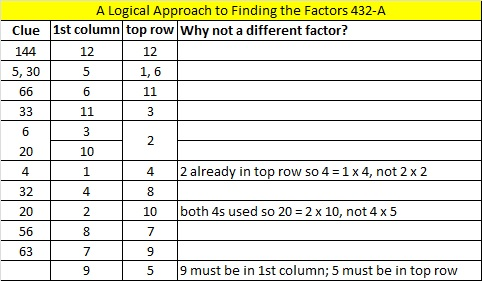# 204 and Level 4

• 204 is a composite number.
• Prime factorization: 204 = 2 × 2 × 3 × 17, which can be written 204 = 2² × 3 × 17
• The exponents in the prime factorization are 2, 1, and 1. Adding one to each and multiplying we get (2 + 1)(1 + 1)(1 + 1) = 3 x 2 x 2 = 12. Therefore 204 has exactly 12 factors.
• Factors of 204: 1, 2, 3, 4, 6, 12, 17, 34, 51, 68, 102, 204
• Factor pairs: 204 = 1 x 204, 2 x 102, 3 x 68, 4 x 51, 6 x 34, or 12 x 17
• Taking the factor pair with the largest square number factor, we get √204 = (√4)(√51) = 2√51 ≈ 14.2828568570856999959987996——————————————————————

Excel file of puzzles and previous week’s factor solutions: 12 Factors 2014-08-11